Courses

# Explanation and Solved Examples - Rectangular Solids GMAT Notes | EduRev

## Quantitative Aptitude for GMAT

Created by: Wizius Careers

## GMAT : Explanation and Solved Examples - Rectangular Solids GMAT Notes | EduRev

The document Explanation and Solved Examples - Rectangular Solids GMAT Notes | EduRev is a part of the GMAT Course Quantitative Aptitude for GMAT.
All you need of GMAT at this link: GMAT

Rectangular Solids
Also known as just a box or a brick, these are pretty straightforward.  Let’s say a solid has a length L, a width W, and a height H.  The volume, of course, is V = LWH.  The surface area is a little trickier: the solid has two faces that are L x W, two that are L x H, and two that are W x H, for a grand total of: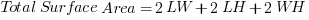For the special case of a cube with side s, the volume is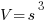, and the surface area simplifies to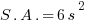What’s a little more subtle about these: the three-dimensional version of the Pythagorean Theorem.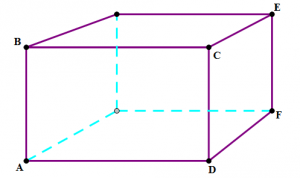Let’s say that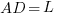,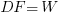, and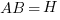.  Lengths like AC, DE, or BE are called “face diagonals”, and to find them, you would just use the ordinary Pythagorean Theorem with the sides of the relevant rectangle.  If we were, though, to construct segment AE, that would go through the empty center of the solid.  In geometry, that’s called a “space diagonal.”  You don’t need to know that vocabulary, but you need to recognize this when it appears, and you need to know you can apply the three-dimensional version of the Pythagorean Theorem.  Let’s say that AE has a length of D.
Then: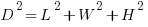Occasionally, a GMAT math problem will ask you to use this formula to evaluate the length of a space diagonal of a rectangular solid.

Cylinders
Cylinders are very familiar from everyday life: a circle at the bottom, a congruent circle directly above it, and smooth curving side (the lateral face) joining one circle to the other. The volume is simply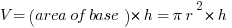.
The surface area is a little trickier.  The top = the bottom = a circle =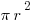, that’s not hard.  What’s tricky is the lateral surface area, the sides of the cylinder.  Think of a can of soup.  In particular, think about the label, which nicely covers the lateral surface of the can.  Suppose we make a vertical cut in that label, and unfurl it to lay it flat.  We get a rectangle.  The height of the rectangle is simply h, the height of the cylinder itself.  The width of the rectangle wrapped all the way around the can before we unfurled it. One way to think about it: it ran around the edge of the circles at the top & bottom.  Well, that length around the edge of a circle —- that’s called the circumference, and its formula is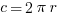.  So, the label is rectangle with length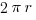and height h, so its area is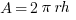.  That’s the formula for the lateral surface area of a cylinder.  To remember that, remember the soup can.

The total surface area is simply that plus the top & bottom circles.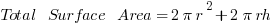Practice Questions

1) K is a rectangular solid.  Find the volume of K
(1) a diagonal line across the front face of K has a length of 40
(2) a diagonal line across the bottom face of K has a length of 25

(A) Statement 1 alone is sufficient but statement 2 alone is not sufficient to answer the question asked.
(B) Statement 2 alone is sufficient but statement 1 alone is not sufficient to answer the question asked.
(C) Both statements 1 and 2 together are sufficient to answer the question but neither statement is sufficient alone.
(D) Each statement alone is sufficient to answer the question.

Ans: E
Solution:
(1) So, the prompt gives us relatively little information.  We are looking for the volume. Statement #1 tells us a diagonal line across the front face has a length of 40.
If L is the length and H is the height, then this means: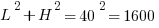.  That’s one equation with two unknowns, and no way to solve for the volume.  Statement #1 by itself is insufficient.
Statement #2 tells us a diagonal line across the bottom face has a length of 25.  If L is the length and W is the width, then this means: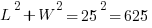.  Again, that’s one equation with two unknowns, and no way to solve for the volume.  Statement #2 by itself is insufficient.
Combine the statements: we now know bothand.  We now have two equations but three unknowns: still not enough information to solve for the individual values, nor can we somehow rearrange to get the product of LWH.  Even combined, the statements are insufficient.

2) M is a rectangular solid.  Find the volume of M
(1) The bottom face of M has an area of 28, and the front face, an area of 35.
(2) All three dimensions of M are positive integers greater than one.

(A) Statement 1 alone is sufficient but statement 2 alone is not sufficient to answer the question asked.
(B) Statement 2 alone is sufficient but statement 1 alone is not sufficient to answer the question asked.
(C) Both statements 1 and 2 together are sufficient to answer the question but neither statement is sufficient alone.
(D) Each statement alone is sufficient to answer the question.

Ans: C
Solution:
Again, a sparse prompt, and we need to find the volume.
Statement #1 tells us the area of the bottom — so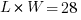, and the area of the front, so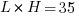.  With this statement alone, we have no idea what the individual dimensions could be.  There’s no guarantee that they are integers: for example,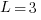,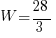, and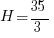satisfy these conditions.  The individual dimensions could be almost anything, which means the volume could be almost anything.  Statement #1by itself is insufficient.
Statement #2 tells us each individual dimension is an integer greater than one, but other than that, we have zero information.  This could be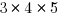, or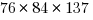.
The volume will be a positive integer, but beyond that, we can determine nothing about it.  Statement #2by itself is insufficient.Combine the statements.  Now we knowand, and that L, W, and H are all integers greater than one.  Well, 28 and 35 have only one common factor greater than one, and that’s 7.   That must mean, L = 7, which means W = 4 and H = 5, which means we could calculate the volume.  Combined, the statements are sufficient.Answer = C

3) Q is a cube.  Find the volume of the cube.
(1)  The total surface area of Q is 150 sq cm
(2) The distance from one vertex of Q to the catty-corner opposite vertex is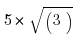(A) Statement 1 alone is sufficient but statement 2 alone is not sufficient to answer the question asked.
(B) Statement 2 alone is sufficient but statement 1 alone is not sufficient to answer the question asked.
(C) Both statements 1 and 2 together are sufficient to answer the question but neither statement is sufficient alone.
(D) Each statement alone is sufficient to answer the question.

Ans: D

Solution:
Another simple prompt, and again, we need the volume.
Statement #1 tells us surface area is 150.  Well, for a cube,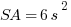.  If we know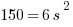, we can solve for s, which will allow us to calculate the volume.  Statement #1 is by itself sufficient.Statement #2 tell us the space diagonal has a length of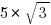.  Well, the three-dimensional version of the Pythagorean Theorem tell us that: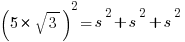That would allow us to solve for s, which would allow us to calculate the volume.

Statement #2 is by itself sufficient.Both statements sufficient by themselves.

4) A certain lighthouse is a tall thin cylinder of brick, with a light chamber at the top.   The brick extends from the ground to the floor of the light chamber. The brick siding of lighthouse is in need of painting.  How many square feet of brick does it have?
(1) The outer diameter of the brick portion of the lighthouse is 28 ft
(2) There are 274 steps from ground level to the floor of the light chamber, and each one is 8 inches high.

(A) Statement 1 alone is sufficient but statement 2 alone is not sufficient to answer the question asked.
(B) Statement 2 alone is sufficient but statement 1 alone is not sufficient to answer the question asked.
(C) Both statements 1 and 2 together are sufficient to answer the question but neither statement is sufficient alone.
(D) Each statement alone is sufficient to answer the question.

Ans: C
Solution:

The lighthouse is essentially a cylinder, and the area that needs to be painted is the lateral surface area. We would need both r and h to calculate this.
Statement #1 tells us diameter = 28, which means radius = 14.  We know the radius, but not the height.  Statement #1 by itself is insufficient.
In Statement #2, the 274 steps go from the very bottom to the very top of the cylinder in question.  We know each step is 8 inches, so the total height traversed by the steps is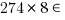.  We would have to change that result from inches to feet, but essentially, with this piece of information, we have the height.  We know the height, but not the radius. Statement #2 by itself is insufficient.
Combined.   Statement #1 gives us the radius, statement #2 gives us the height, and we’re in business!  We now have enough information to calculate the lateral surface area.  Combined, the statements are sufficient.

Offer running on EduRev: Apply code STAYHOME200 to get INR 200 off on our premium plan EduRev Infinity!

## Quantitative Aptitude for GMAT

85 videos|100 docs|176 tests

,

,

,

,

,

,

,

,

,

,

,

,

,

,

,

,

,

,

,

,

,

;